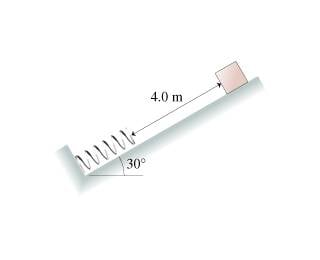# Mass on incline sliding into spring, how compressed?

## Homework Statement

A 14 kg box slides 4.0 {\rm m} down the frictionless ramp shown in the figure , then collides with a spring whose spring constant is 210 N/m.a)What is the maximum compression of the spring?

b)At what compression of the spring does the box have its maximum speed?

## Homework Equations

PEspring = (1/2)k(s)^2
Fsp = -ks

## The Attempt at a Solution

I thought this was a simple conversion of potential energy to a springs potential energy. So I tried mgh = (1/2)ks^2 where h=4sin(30). That gets me s=1.6 which is not correct. Do you have to take into account the force of gravity on the spring because it is not horizontal?

I haven't started the second part but I believe you would use a free body diagram, and the maximum speed is when the force of the spring exactly equals the force of gravity, i.e. when acceleration=0.

Thanks so much for any help!

## The Attempt at a Solution

collinsmark
Homework Helper
Gold Member
I thought this was a simple conversion of potential energy to a springs potential energy. So I tried mgh = (1/2)ks^2 where h=4sin(30). That gets me s=1.6 which is not correct. Do you have to take into account the force of gravity on the spring because it is not horizontal?

Sort of, but not the spring itself. Consider that the total vertical distance that the mass travels. It is not just 4sin(30) meters. Once the mass hits the spring, the spring compresses by amount s. So the mass also travels a little more verically by the sin(30) component of s. Take the entire horizontal displacement when calculating the gravitational potential energy. Then set that equal to (1/2)ks2.Thanks so much, that makes a lot of sense

i still don't understand what you mean by taking the whole horizontal distance into account...

collinsmark
Homework Helper
Gold Member
i still don't understand what you mean by taking the whole horizontal distance into account...

Oops. I mistyped. I meant take the whole vertical distance into account. Silly me.